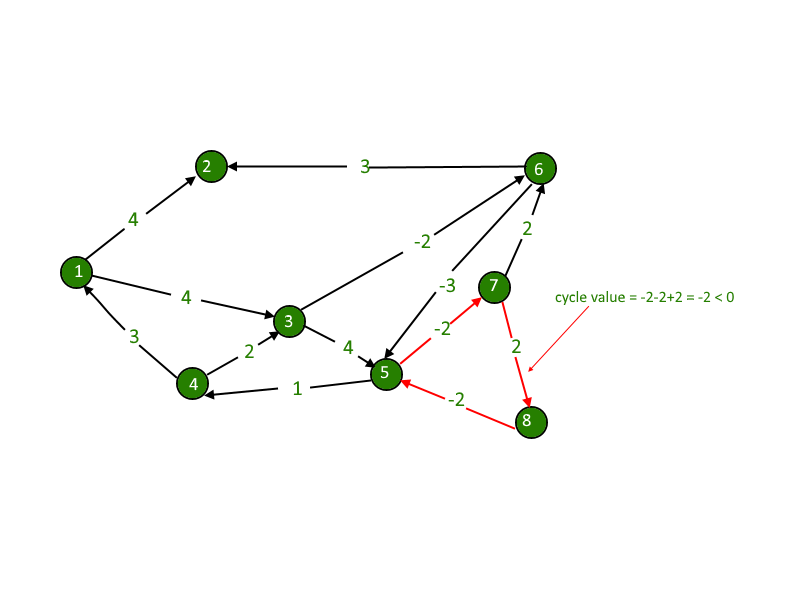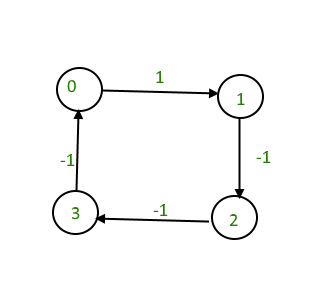# Detecting negative cycle using Floyd Warshall

• Difficulty Level : Easy
• Last Updated : 20 Jul, 2022

We are given a directed graph. We need compute whether the graph has negative cycle or not. A negative cycle is one in which the overall sum of the cycle comes negative.Negative weights are found in various applications of graphs. For example, instead of paying cost for a path, we may get some advantage if we follow the path.

Examples:

```Input : 4 4
0 1 1
1 2 -1
2 3 -1
3 0 -1

Output : Yes
The graph contains a negative cycle.```` `

We have discussed Bellman Ford Algorithm based solution for this problem.
In this post, Floyd Warshall Algorithm based solution is discussed that works for both connected and disconnected graphs.
Distance of any node from itself is always zero. But in some cases, as in this example, when we traverse further from 4 to 1, the distance comes out to be -2, i.e. distance of 1 from 1 will become -2. This is our catch, we just have to check the nodes distance from itself and if it comes out to be negative, we will detect the required negative cycle.

Implementation:

## C++

 `// C++ Program to check if there is a negative weight``// cycle using Floyd Warshall Algorithm``#include``using` `namespace` `std;` `// Number of vertices in the graph``#define V 4`` ` `/* Define Infinite as a large enough value. This``   ``value will be used for vertices not connected``   ``to each other */``#define INF 99999`` ` `// A function to print the solution matrix``void` `printSolution(``int` `dist[][V]);`` ` `// Returns true if graph has negative weight cycle``// else false.``bool` `negCyclefloydWarshall(``int` `graph[][V])``{``    ``/* dist[][] will be the output matrix that will``       ``finally have the shortest``    ``distances between every pair of vertices */``    ``int` `dist[V][V], i, j, k;`` ` `    ``/* Initialize the solution matrix same as input``      ``graph matrix. Or we can say the initial values``      ``of shortest distances are based on shortest``      ``paths considering no intermediate vertex. */``    ``for` `(i = 0; i < V; i++)``        ``for` `(j = 0; j < V; j++)``            ``dist[i][j] = graph[i][j];`` ` `    ``/* Add all vertices one by one to the set of``       ``intermediate vertices.``    ``---> Before start of a iteration, we have shortest``         ``distances between all pairs of vertices such``         ``that the shortest distances consider only the``         ``vertices in set {0, 1, 2, .. k-1} as intermediate``         ``vertices.``    ``----> After the end of a iteration, vertex no. k is``          ``added to the set of intermediate vertices and``          ``the set becomes {0, 1, 2, .. k} */``    ``for` `(k = 0; k < V; k++)``    ``{``        ``// Pick all vertices as source one by one``        ``for` `(i = 0; i < V; i++)``        ``{``            ``// Pick all vertices as destination for the``            ``// above picked source``            ``for` `(j = 0; j < V; j++)``            ``{``                ``// If vertex k is on the shortest path from``                ``// i to j, then update the value of dist[i][j]``                ``if` `(dist[i][k] + dist[k][j] < dist[i][j])``                        ``dist[i][j] = dist[i][k] + dist[k][j];``            ``}``        ``}``    ``}` `    ``// If distance of any vertex from itself``    ``// becomes negative, then there is a negative``    ``// weight cycle.``    ``for` `(``int` `i = 0; i < V; i++)``        ``if` `(dist[i][i] < 0)``            ``return` `true``;``    ``return` `false``;``}`` ` ` ``// driver program``int` `main()``{``    ``/* Let us create the following weighted graph``            ``1``    ``(0)----------->(1)``    ``/|\             |``     ``|              |``  ``-1 |              | -1``     ``|             \|/``    ``(3)<-----------(2)``        ``-1       */``        ` `    ``int` `graph[V][V] = { {0   , 1   , INF , INF},``                        ``{INF , 0   , -1  , INF},``                        ``{INF , INF , 0   ,  -1},``                        ``{-1  , INF , INF ,   0}};``    ` `    ``if` `(negCyclefloydWarshall(graph))``       ``cout << ``"Yes"``;``    ``else``       ``cout << ``"No"``;``    ``return` `0;``}`

## Java

 `// Java Program to check if there is a negative weight``// cycle using Floyd Warshall Algorithm``class` `GFG``{` `    ``// Number of vertices in the graph``    ``static` `final` `int` `V = ``4``;``    ` `    ``/* Define Infinite as a large enough value. This``    ``value will be used for vertices not connected``    ``to each other */``    ``static` `final` `int` `INF = ``99999``;``    ` `    ``// Returns true if graph has negative weight cycle``    ``// else false.``    ``static` `boolean` `negCyclefloydWarshall(``int` `graph[][])``    ``{``        ` `        ``/* dist[][] will be the output matrix that will``        ``finally have the shortest``        ``distances between every pair of vertices */``        ``int` `dist[][] = ``new` `int``[V][V], i, j, k;``    ` `        ``/* Initialize the solution matrix same as input``        ``graph matrix. Or we can say the initial values``        ``of shortest distances are based on shortest``        ``paths considering no intermediate vertex. */``        ``for` `(i = ``0``; i < V; i++)``            ``for` `(j = ``0``; j < V; j++)``                ``dist[i][j] = graph[i][j];``    ` `        ``/* Add all vertices one by one to the set of``        ``intermediate vertices.``        ``---> Before start of a iteration, we have shortest``            ``distances between all pairs of vertices such``            ``that the shortest distances consider only the``            ``vertices in set {0, 1, 2, .. k-1} as intermediate``            ``vertices.``        ``----> After the end of a iteration, vertex no. k is``            ``added to the set of intermediate vertices and``            ``the set becomes {0, 1, 2, .. k} */``        ``for` `(k = ``0``; k < V; k++)``        ``{``            ` `            ``// Pick all vertices as source one by one``            ``for` `(i = ``0``; i < V; i++)``            ``{``                ` `                ``// Pick all vertices as destination for the``                ``// above picked source``                ``for` `(j = ``0``; j < V; j++)``                ``{``                    ` `                    ``// If vertex k is on the shortest path from``                    ``// i to j, then update the value of dist[i][j]``                    ``if` `(dist[i][k] + dist[k][j] < dist[i][j])``                            ``dist[i][j] = dist[i][k] + dist[k][j];``                ``}``            ``}``        ``}``    ` `        ``// If distance of any vertex from itself``        ``// becomes negative, then there is a negative``        ``// weight cycle.``        ``for` `(i = ``0``; i < V; i++)``            ``if` `(dist[i][i] < ``0``)``                ``return` `true``;` `        ``return` `false``;``    ``}``        ` `    ``// Driver code``    ``public` `static` `void` `main (String[] args)``    ``{``    ` `    ``/* Let us create the following weighted graph``                ``1``        ``(0)----------->(1)``        ``/|\               |``         ``|               |``      ``-1 |               | -1``         ``|                \|/``        ``(3)<-----------(2)``            ``-1     */``            ` `        ``int` `graph[][] = { {``0``, ``1``, INF, INF},``                          ``{INF, ``0``, -``1``, INF},``                          ``{INF, INF, ``0``, -``1``},``                          ``{-``1``, INF, INF, ``0``}};``        ` `        ``if` `(negCyclefloydWarshall(graph))``            ``System.out.print(``"Yes"``);``        ``else``            ``System.out.print(``"No"``);``    ``}``}` `// This code is contributed by Anant Agarwal.`

## Python3

 `# Python Program to check``# if there is a``# negative weight``# cycle using Floyd``# Warshall Algorithm` ` ` `# Number of vertices``# in the graph``V ``=` `4``     ` `# Define Infinite as a``# large enough value. This``# value will be used``#for vertices not connected``# to each other``INF ``=` `99999``     ` `# Returns true if graph has``# negative weight cycle``# else false.``def` `negCyclefloydWarshall(graph):``         ` `    ``# dist[][] will be the``    ``# output matrix that will``    ``# finally have the shortest``    ``# distances between every``    ``# pair of vertices``    ``dist``=``[[``0` `for` `i ``in` `range``(V``+``1``)]``for` `j ``in` `range``(V``+``1``)]``     ` `    ``# Initialize the solution``    ``# matrix same as input``    ``# graph matrix. Or we can``    ``# say the initial values``    ``# of shortest distances``    ``# are based on shortest``    ``# paths considering no``    ``# intermediate vertex.``    ``for` `i ``in` `range``(V):``        ``for` `j ``in` `range``(V):``            ``dist[i][j] ``=` `graph[i][j]``     ` `    ``''' Add all vertices one``        ``by one to the set of``        ``intermediate vertices.``    ``---> Before start of a iteration,``         ``we have shortest``        ``distances between all pairs``        ``of vertices such``        ``that the shortest distances``        ``consider only the``        ``vertices in set {0, 1, 2, .. k-1}``        ``as intermediate vertices.``    ``----> After the end of a iteration,``          ``vertex no. k is``        ``added to the set of``        ``intermediate vertices and``        ``the set becomes {0, 1, 2, .. k} '''``    ``for` `k ``in` `range``(V):``    ` `        ``# Pick all vertices``        ``# as source one by one``        ``for` `i ``in` `range``(V):``                 ` `            ``# Pick all vertices as``            ``# destination for the``            ``# above picked source``            ``for` `j ``in` `range``(V):``        ` `                ``# If vertex k is on``                ``# the shortest path from``                ``# i to j, then update``                ``# the value of dist[i][j]``                ``if` `(dist[i][k] ``+` `dist[k][j] < dist[i][j]):``                        ``dist[i][j] ``=` `dist[i][k] ``+` `dist[k][j]`` ` `    ``# If distance of any``    ``# vertex from itself``    ``# becomes negative, then``    ``# there is a negative``    ``# weight cycle.``    ``for` `i ``in` `range``(V):``        ``if` `(dist[i][i] < ``0``):``            ``return` `True`` ` `    ``return` `False` `         ` `# Driver code` `     ` `''' Let us create the``    ``following weighted graph``            ``1``    ``(0)----------->(1)``    ``/|\               |``     ``|               |``  ``-1 |               | -1``     ``|                \|/``    ``(3)<-----------(2)``        ``-1     '''``         ` `graph ``=` `[ [``0``, ``1``, INF, INF],``          ``[INF, ``0``, ``-``1``, INF],``          ``[INF, INF, ``0``, ``-``1``],``          ``[``-``1``, INF, INF, ``0``]]``         ` `if` `(negCyclefloydWarshall(graph)):``    ``print``(``"Yes"``)``else``:``    ``print``(``"No"``)` `# This code is contributed``# by Anant Agarwal.`

## C#

 `// C# Program to check if there``// is a negative weight cycle``// using Floyd Warshall Algorithm` `using` `System;` `namespace` `Cycle``{``public` `class` `GFG``{    ``    `  `    ``// Number of vertices in the graph``    ``static` `int` `V = 4;``    ` `    ``/* Define Infinite as a large enough value. This``    ``value will be used for vertices not connected``    ``to each other */``    ``static` `int` `INF = 99999;``    ` `    ``// Returns true if graph has negative weight cycle``    ``// else false.``    ``static` `bool` `negCyclefloydWarshall(``int` `[,]graph)``    ``{``        ` `        ``/* dist[][] will be the output matrix that will``        ``finally have the shortest``        ``distances between every pair of vertices */``        ``int` `[,]dist = ``new` `int``[V,V];``        ``int` `i, j, k;``    ` `        ``/* Initialize the solution matrix same as input``        ``graph matrix. Or we can say the initial values``        ``of shortest distances are based on shortest``        ``paths considering no intermediate vertex. */``        ``for` `(i = 0; i < V; i++)``            ``for` `(j = 0; j < V; j++)``                ``dist[i,j] = graph[i,j];``    ` `        ``/* Add all vertices one by one to the set of``        ``intermediate vertices.``        ``---> Before start of a iteration, we have shortest``            ``distances between all pairs of vertices such``            ``that the shortest distances consider only the``            ``vertices in set {0, 1, 2, .. k-1} as intermediate``            ``vertices.``        ``----> After the end of a iteration, vertex no. k is``            ``added to the set of intermediate vertices and``            ``the set becomes {0, 1, 2, .. k} */``        ``for` `(k = 0; k < V; k++)``        ``{``            ` `            ``// Pick all vertices as source one by one``            ``for` `(i = 0; i < V; i++)``            ``{``                ` `                ``// Pick all vertices as destination for the``                ``// above picked source``                ``for` `(j = 0; j < V; j++)``                ``{``                    ` `                    ``// If vertex k is on the shortest path from``                    ``// i to j, then update the value of dist[i][j]``                    ``if` `(dist[i,k] + dist[k,j] < dist[i,j])``                            ``dist[i,j] = dist[i,k] + dist[k,j];``                ``}``            ``}``        ``}``    ` `        ``// If distance of any vertex from itself``        ``// becomes negative, then there is a negative``        ``// weight cycle.``        ``for` `(i = 0; i < V; i++)``            ``if` `(dist[i,i] < 0)``                ``return` `true``;` `        ``return` `false``;``    ``}``        ` `    ``// Driver code``    ``public` `static` `void` `Main()``    ``{``    ` `    ``/* Let us create the following weighted graph``                ``1``        ``(0)----------->(1)``        ``/|\             |``        ``|             |``    ``-1 |             | -1``        ``|             \|/``        ``(3)<-----------(2)``            ``-1     */``            ` `        ``int` `[,]graph = { {0, 1, INF, INF},``                        ``{INF, 0, -1, INF},``                        ``{INF, INF, 0, -1},``                        ``{-1, INF, INF, 0}};``        ` `        ``if` `(negCyclefloydWarshall(graph))``            ``Console.Write(``"Yes"``);``        ``else``            ``Console.Write(``"No"``);``    ``}``}``}` `// This code is contributed by Sam007.`

## Javascript

 ``

Output

`Yes`

This article is contributed by Shivani Mittal. If you like GeeksforGeeks and would like to contribute, you can also write an article using write.geeksforgeeks.org or mail your article to review-team@geeksforgeeks.org. See your article appearing on the GeeksforGeeks main page and help other Geeks.

My Personal Notes arrow_drop_up